Leak Rate Calculator

Leak rate for liquid in pipe or tank

Register to enable "Calculate" button.

 Select liquid: Water at 20 C (68 F) Seawater at 16 C (60 F) Diesel at 15 C (59 F) SAE 30 oil at 16 C (60 F) Gasoline at 16 C (60 F) User Enters S © 2017 LMNO Engineering, Select crack geometry: Circular Non-circular Research, and Software, Ltd. Select computation: Compute Leak Rate Compute Pressure Drop Compute Hole Area Specific Gravity, S: Discharge Coefficient, C: www.LMNOeng.com Crack Diameter, d: mm cm m inch ft Crack Area, A: mm2 cm2 m2 inch2 ft2 Pressure Drop, ΔP: mm Mercury (0C) mbar N/m2 (Pa) kPa MPa inch H2O (60F) inch Mercury (60F) kg/cm2 lb/in2 (psi) lb/ft2 (psf) Leak Rate, Q: m3/s m3/min m3/hr m3/day ft3/s ft3/min ft3/hr ft3/day gal/min (US) gal/hr (US) gal/day (US) Velocity, V: m/s ft/s

Water Leak from Hole in Copper Pipe (photograph)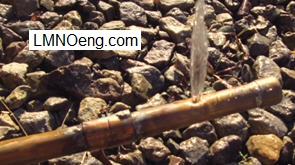Leak Rate Equations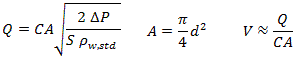Leak Rate
Leaks are common occurrences in pipes, tubes, and tanks. The leak rate is based on the pressure difference between the inside and outside of the pipe, the crack or hole area, and specific gravity of the liquid. The pipe leak rate calculator computes the leak rate of a liquid out of a circular or non-circular crack or hole in a pipe or tank. The leak rate calculation has built-in specific gravity values for water, seawater, diesel fuel, SAE 30 oil, and gasoline. Specific gravity can be entered for other liquids. If the leak rate is known, the calculator can compute pressure or crack area (and diameter).

Leak Rate Equations and Derivation

Liquid inside a pipe, tank, or other confined space flows out through a hole or crack. The liquid in the pipe is at an elevation Z1, has pressure P1, and has velocity V1. As the liquid exits the hole, it increases to velocity V2 through the hole, pressure P2 at the hole exit, and an elevation Z2 of the hole exit.

Diagram of liquid flowing out of pipe through crack: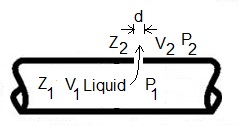The Bernoulli equation (Munson et al., 1998, p. 114; bernoulli.php) describes flow of an inviscid fluid flowing from location 1 to location 2.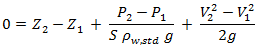Specific gravity S = ρ/ρw,std, the ratio of density of the flowing liquid to density of water at standard conditions (4oC), which is 1000 kg/m3.

The Bernoulli equation has many applications. Leak rate is one application where it is widely used. Since a pipe is usually much larger diameter than the hole, V2>>V1. Since the elevation change Z2-Z1 is typically very small compared to other terms in the Bernoulli equations for a pipe leak application, Z2-Z1=0. Thus: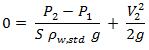Solve for V2: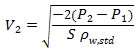Then with Q=V2A2, A=πd2 / 4, and ΔP=P1-P2: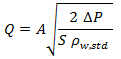Due to the viscosity of liquids and the tortuous path of the liquid through the hole, the measured leak rate from a hole is less that that computed by the above equation. To account for these effects, a discharge coefficient C is commonly used in the equation (e.g. Munson et al., 1998, p. 531; McAllister, 2005, p. 356):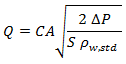C=0.61 is a typical value determined from experiments (McAllister, 2005, p. 356). Velocity out of the hole is computed approximately as: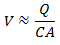A more accurate computation of velocity can be found using the methods at our Discharge from a Tank calculation which splits C into a velocity coefficient and a contraction coefficient. Our Bernoulli equation calculator applies the Bernoulli equation to many situations including pipe leak, but does not include a discharge coefficient.

Variables
The units shown for the variables are SI (International System of Units); however, the equations above are valid for any consistent set of units. Our calculation allows a variety of units; all unit conversions are accomplished internally.
A = Hole or crack area (m2).
C = Discharge coefficient. Need 0 < C ≤ 1. Suggested value is C=0.61 (McAllister, 2005, p. 356). For highly viscous liquids, C is lower.
d = Hole diameter (m).
g = Acceleration due to gravity, 9.8066 m/s2.
Q = Leak rate (m3/s).
S = Liquid specific gravity. In calculation, S for water is from Munson et al. (1998, p. B.2); seawater, SAE 30 oil, and gasoline are from Munson Table 1.6; and diesel is from Chevron (2007, p. 48).
V = Leak velocity (m/s).
ΔP = Pressure drop from inside of pipe to outside of pipe (or tank) (N/m2).
ρw,std = Mass density of water at standard conditions (4oC), 1000 kg/m3.
π = 3.1415926...

Error Messages given by calculation
"Need A > 0", "Need 0 < C ≤ 1", "Need d > 0", "Need S > 0", "Need Q > 0", "Need ΔP > 0".

References

McAllister, E. W. (editor). 2005. Pipeline Rules of Thumb Handbook. Elsevier - Gulf Professional Publishing.

Munson, B.R., Young, D.F, and Okiishi, T. H. 1998. Fundamentals of Fluid Mechanics. John Wiley and Sons, Inc. 3ed.

LMNO Engineering, Research, and Software, Ltd.
7860 Angel Ridge Rd.   Athens, Ohio 45701  USA   Phone: (740) 707-2614
LMNO@LMNOeng.com    https://www.LMNOeng.com

To:

Related calculations:

Bernoulli applications

Time to drain a tank

Discharge from a tank (steady state)

Orifice flow meter in pipe for liquids (D<5cm)

Orifice flow meter in pipe for liquids (D5cm)

Other information:

Unit Conversions Calculator

Register